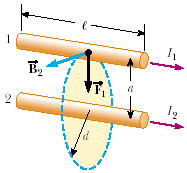# Problem: If, in the figure below, I1 = 2 A and I2 = 6 A, which of the following is true?1. F1 = 3 F2   2. F1 = F2/33. F1 = F2

###### FREE Expert Solution

This force is based on the force between parallel current-carrying conductors.

79% (420 ratings)###### Problem Details

If, in the figure below, I1 = 2 A and I2 = 6 A, which of the following is true?1. F1 = 3 F2

2. F1 = F2/3

3. F= F2